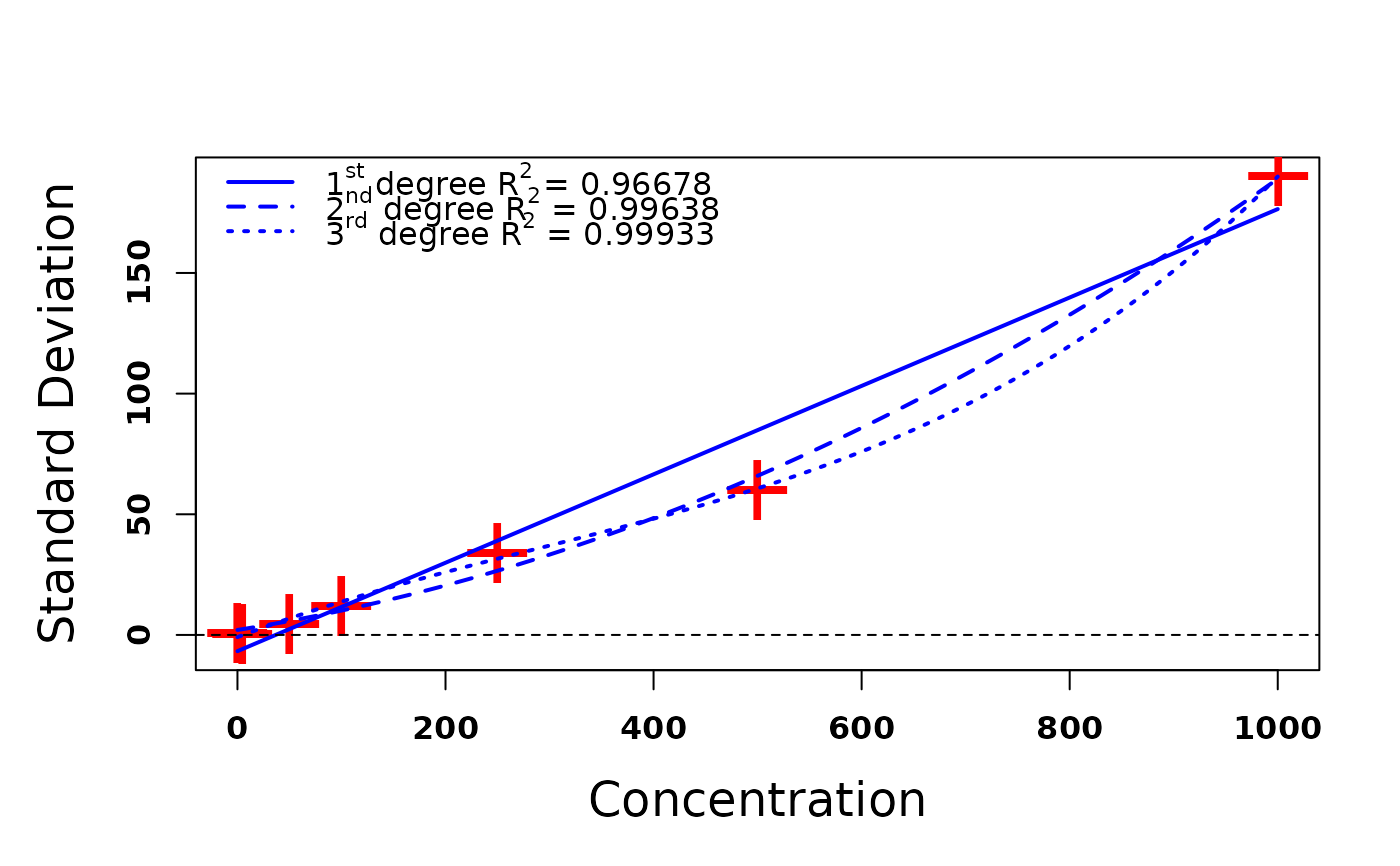Estimate coefficients for the polynomial to describe assay error.

## Usage

makeErrorPoly(
obs,
sd,
data,
outeq = 1,
col = "red",
cex = 3,
pch = "+",
lcol = "blue",
lwd = 2,
ref = T,
legend = T,
...
)

## Arguments

obs

A vector of observations

sd

A vector of standard deviations obtained from repeated measurements at each observation in obs

data

A Pmetrics data file. From this, the maximum and mininimum observations will be retrieved. This is useful to ensure that calculated standard deviations are not negative at any observation in the dataset. If not specified, the default is the maximum obs.

outeq

The output equation in data. Default is 1.

col

Color of the data points. Default is red.

cex

Relative size of the data points. Default is 3. See par.

pch

Ploting symbol. Default is “+”. See par.

lcol

Color of the fitted polynomial lines. Default is blue.

lwd

Width of the lines. Default is 2.

ref

Add a reference line at SD 0 to help evaluate that all fitted SDs are >0. Default is true.

legend

Boolean argument to plot legend. Default is TRUE.

...

Other plotting parameters as in plot.default and par

## Value

A plot of the measured observations and fitted polynomial curves and a list with the first, second, and third order coefficients

## Details

This function plots first, second, and third order polynomial functions fitted to pairs of observations and associated standard deviations for a given output assay. In this way, the standard deviation associated with any observation may be calculated and used to appropriately weight that observation in the model building process. Observations are weighted by the reciprocal of the variance, or squared standard deviation.

Michael Neely

## Examples

makeErrorPoly(obs = c(0,5,50,100,250,500,1000), sd = c(1,0.4,4.5,12,34,60,190))#> $first #> C0 C1 #> -6.7277503 0.1831991 #> #>$second
#>           C0           C1           C2
#> 2.0608389240 0.0685990444 0.0001183321
#>
#> \$third
#>            C0            C1            C2            C3
#> -9.004376e-01  1.636062e-01 -1.886027e-04  2.158411e-07
#>